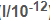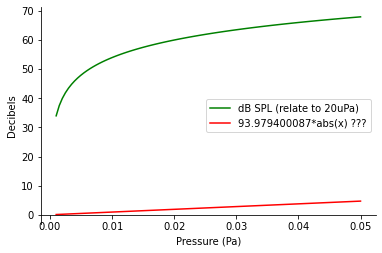# SWARM 'Power' unit ? and dB equivalence?

Hi all,

In SWARM graphics, the power is expressed as powers of 10. But I don’t see any unit.
Is it watts or watts/meter?

If I’d like to convert to dB (not dbA), should I use the following formula?(10Log10(I/10-12)), where I is Intensity in watts/meter.

Or is it directly the ratio? so no unit makes sense… Then dB would only be the power number x 10.

I don’t know much about sound. Sorry if that’s a naive question…

Hello vaudoin,

if I remember well you have a RBOOM, so this can be useful to convert the counts output in Pascal and then in Decibels:

https://manual.raspberryshake.org/developersCorner.html#converting-counts-to-pascal-to-decibel-using-obspy

You need to have basic knowledge of Python language, however.

For the SWARM question, have you consulted its manual? Here’s the link: https://volcanoes.usgs.gov/software/swarm/doc/swarm_v3.pdf

Thank you @Stormchaser!

Alright, I’ll have to code a bit thenI’ve already checked the SWARM manual but nothing is mentioned about the power unit or ratio. Only found this:

The y-axis of the spectra plot is the ‘power’ or ‘amplitude’ of the frequency that contributes to the actual signal. Actual value is often less important than the relative value.

So I guess the Spectra chart Power axis is giving just an idea of the amplitude per frequency distribution but is not accurate for a dB perspective.
(As I am focusing on periodic events - wind turbines - with less power than earthquakes, the Spectra is useful!)

For RB the SWARM programs does not do anything about the instrument response at all. If you say “use calibrations” it will display pascals, but actually give you counts.

The python programs will take into account the calibration and instrument response but give you amplitude as a function of time over the whole passband. So you cannot say what the magnitudes of the various frequencies are. There may be a python function for filtering. It seems like a pretty powerful package, but quite a bit of work to get set up.

In some ways RB is simpler than RS. RS gives you velocity but what people are often interested in is acceleration or displacement. RB gives you (sound) pressure.directly and that is what is usually of interest.

There is a direct relationship between sound power (intensity) and sound pressure level. It is usually expressed in dB relative to 10^-12 watts/m^2.

Sound Intensity = (Sound Pressure)2 / 400

There is no explanation of what SWARM does about power. Hopefully they are squaring the readings and dividing by something …

Thank you again Ken @kpjamro to lignthen this.

I am going forward with Python…
@Stormchaser:
On my steps, I found that on the samples for developpers to convert counts to Pascals to Decibels (Developer’s corner — Instructions on Setting Up Your Raspberry Shake) the calculation from pascal to decibal looks weird:

93.979400087*abs(st.data/56000)

As I understand it should be:

import numpy as np
20*np.log10(abs(st.data/56000)/0.00002)I also checked with the conversion liked given by Ken : Convert pascal [Pa] to sound pressure level in decibels [dB SPL] • Sound Pressure Level (SPL) Converter • Acoustics — Sound • Compact Calculator • Online Unit Converters

Or I missed something…

Hello @vaudoin,

thank you for pointing that out, it is indeed as you say. The example in the developer’s guide offered a linear approximation, to better highlight the impact that the pressure variation caused by the thunder made on the RBOOM sensor.

I will add a section with the formula you indicated, which represents the precise logarithmic approach to the Pascals to Decibels conversion.

2 Likes